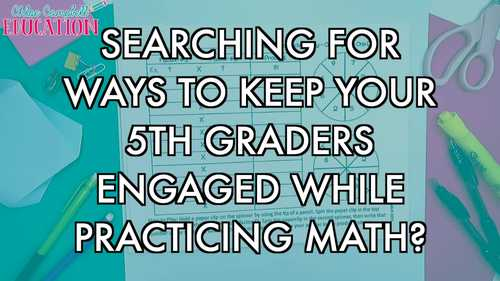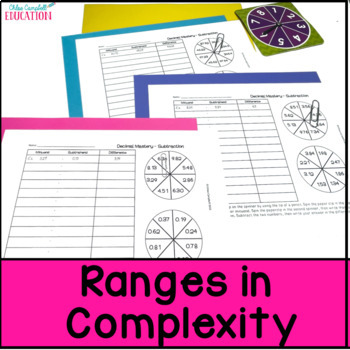# Adding and Subtracting Decimals | Multiplying and Dividing Decimals Games BUNDLE

Rated 5 out of 5, based on 12 reviews
12 Ratings;
4th - 6th
Subjects
Resource Type
Standards
Formats Included
• Zip
Pages
28 pages
\$5.20
List Price:
\$12.00
Bundle Price:
\$6.50
You Save:
\$6.80
Bundle
\$5.20
List Price:
\$12.00
Bundle Price:
\$6.50
You Save:
\$6.80
Bundle
Report this resource to TPT

#### Products in this Bundle (4)

##### Also included in
1. Get your students engaged in practicing 5th grade math standards with these fun and effective spin and answer games! This bundle includes no-prep practice activities that are easily differentiated to meet all of your students’ needs. Just add a paperclip to play! Use these games as small group games
Price \$22.40Original Price \$55.25Save \$32.85
2. Are you always searching for engaging stations for specific math skills? You found the perfect 5th grade math centers set for the entire school year! Your 5th grade students will LOVE practicing or reviewing important math concepts with these hands-on and engaging math centers. This year-long bundle
Price \$126.00Original Price \$313.50Save \$187.50

### Description

Practice adding, subtracting, multiplying, and dividing decimals in a fun, hands-on way! Get your students engaged in practicing 5th grade math standards with this fun and effective spin and answer game! This download includes no-prep practice activities that are easily differentiated to meet all of your students’ needs. Just add a paperclip to play! Use these games as small group games, math stations or whole class review. Set up as a fun spinner game, your students won’t even notice that they are completing rigorous math problems.

This add, subtract, multiply, divide decimals spinner math game is the perfect math game for your 4th - 6th grade class. Students will learn and practice all operations with decimals. This printable game is sure to be a crowd favorite for your class. The math game is easy to prep and will provide your students with hours of fun learning. Just print the games, decide the level for each student, add a paperclip, and begin increasing engagement immediately. With games for below level, on level and above level you can quickly and easily differentiate for the needs of your students. Students will practice adding, subtracting, multiplying, and dividing decimals in a fun and engaging way.

This Spin and Answer Math Game Includes:

• Teacher Tips
• Directions Page
• 28 no-prep printable decimals games:
1. Adding Decimals (Tenths + Whole Number)
2. Adding Decimals (Tenths + Tenths)
3. Adding Decimals (Hundredths + Tenths)
4. Adding Decimals (Hundredths + Hundredths)
5. Adding Decimals (Thousandths + Hundredths)
6. Adding Decimals (Thousandths + Thousandths)
7. Adding Decimals (Highest Complexity with Mix of Place Value)
8. Subtracting Decimals (Whole Number - Tenths)
9. Subtracting Decimals (Tenths - Tenths)
10. Subtracting Decimals (Two Digit Whole Number with Tenths - Tenths)
11. Subtracting Decimals (Hundredths - Hundredths)
12. Subtracting Decimals (Hundredths - Hundredths)
13. Subtracting Decimals (Hundredths - Thousandths)
14. Subtracting Decimals (Two Digit Whole Numbers with Hundredths - Hundredths)
15. Multiplying Decimals (Tenths x Whole Number)
16. Multiplying Decimals (Tenths x Tenths)
17. Multiplying Decimals (Hundredths x Tenths)
18. Multiplying Decimals (Hundredths x Hundredths)
19. Multiplying Decimals (Thousandths x Hundredths)
20. Multiplying Decimals (Thousandths x Thousandths)
21. Multiplying Decimals (Hundredths x Thousandths)
22. Dividing Decimals (Tenths / Whole Number)
23. Dividing Decimals (Tenths / Tenths)
24. Dividing Decimals (Hundredths / Tenths)
25. Dividing Decimals (Hundredths / Hundredths)
26. Dividing Decimals (Thousandths / Hundredths)
27. Dividing Decimals (Thousandths / Thousandths)
28. Dividing Decimals (Highest Complexity with Mix of Place Value)

Love this game? Find the whole year bundle here!

How to Play: Students play the game by holding a paperclip on the spinner using the tip of a pencil. They spin the paperclip on the top spinner first and write that number under column #1. Then they spin the paperclip on the second spinner then write that number under column #2. Students use the two numbers to complete the problem and write their answer in the column #3.

What’s the best way to use these spin and answer games?

• Math Centers or Stations
• Whole Group Practice
• Morning Work
• Early Finisher Activities
• Substitutes
• Send home to engage students’ families

Tips for Playing this Math Game:

• Read the directions to the students and model how to play.
• Be prepared with paperclips for each player.
• Every student should solve every problem - not just the person who spins.
• Create groups of 2-4 students. The lower number of students means the more focused students are while playing.
• Remind students that the focus is not playing the game but that’s just an added bonus! The focus should be on practicing math skills.
• Show students how to compare and discuss answers. Did you both get the same answer? If students get different answers, ask them to solve the problem using a different strategy or help coach each other through the problem.

Do your students need math practice? Students will be playing a game and will often forget that they are even practicing math skills! Research shows that challenge-based gamification in the classroom leads to an increase of 34.755% in student performance (ScienceDirect, 2020).

The best part? Your students will quickly learn the instructions to play the spin and answer games and will get right to work on playing (and practicing) their math skills. This resource is easy to use and requires very little prep. It’s also printer friendly so you’ll only use black ink!

Standard Covered:

5.NBT.B.7 Add, subtract, multiply, and divide decimals to hundredths, using concrete models or drawings and strategies based on place value, properties of operations, and/or the relationship between addition and subtraction; relate the strategy to a written method and explain the reasoning used.

Here’s what other teachers have to say about these spin and answer games...

⭐⭐⭐⭐⭐ “What a way to engage students!”

⭐⭐⭐⭐⭐ “Easy to use, my students enjoyed this resource as a math fluency review center.”

⭐⭐⭐⭐⭐ “I love how there are many different ways to use this as differentiation!! Thank you!”

Save money on future purchases!

Go to your My Purchases page (log in first). Click on the Provide Feedback button to leave a rating and a short comment. You’ll earn TPT credits, which you can redeem on future purchases!

You may also like…

Having difficulty with a file?

Visit the FAQs section, submit a help ticket, or ask a question on the Q& A tab before leaving feedback.

Permission to copy for single classroom use only.

Total Pages
28 pages
Does not apply
Teaching Duration
1 month
Report this resource to TPT
Reported resources will be reviewed by our team. Report this resource to let us know if this resource violates TPT’s content guidelines.

### Standards

to see state-specific standards (only available in the US).
Add, subtract, multiply, and divide decimals to hundredths, using concrete models or drawings and strategies based on place value, properties of operations, and/or the relationship between addition and subtraction; relate the strategy to a written method and explain the reasoning used.
Fluently add, subtract, multiply, and divide multi-digit decimals using the standard algorithm for each operation.